#### You may also likeA 1 metre cube has one face on the ground and one face against a wall. A 4 metre ladder leans against the wall and just touches the cube. How high is the top of the ladder above the ground?In this problem we are faced with an apparently easy area problem, but it has gone horribly wrong! What happened?### From All Corners

Straight lines are drawn from each corner of a square to the mid points of the opposite sides. Express the area of the octagon that is formed at the centre as a fraction of the area of the square.

# Triangular Slope

##### Age 14 to 16 Short Challenge Level:

Answer: $\frac 43$ and $-\frac34$

Using scale factors
OA
Red triangles are similar so use scale factor $\frac35=0.6$ to find missing lengths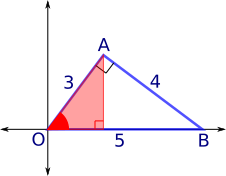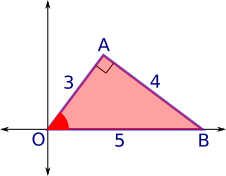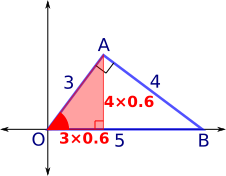Gradient is $\dfrac{4\times0.6}{3\times0.6}=\dfrac43$

AB
Green triangles are similar with scale factor $\frac45=0.8$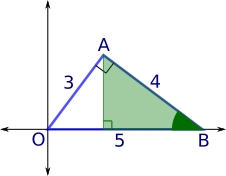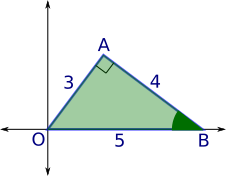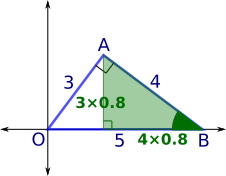Gradient is $\dfrac{-3\times0.6}{4\times0.6}=-\dfrac34$

Using similar and congruent triangles
OA
Red triangles are similar so use large red triangle for the correct ratios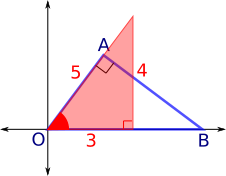Gradient is $\dfrac43$

AB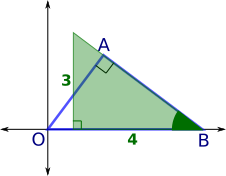Gradient is $\dfrac{-3}{4}=-\dfrac34$

You can find more short problems, arranged by curriculum topic, in our short problems collection.